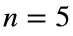# Problem 51282. Compute a row of the Kimberling shuffle

This problem continues from Cody Problem 49803.
The Kimberling shuffle uses a semi-infinite array of numbers in which the first row is simply the numbers 1, 2, 3, 4, 5,… Subsequent rows are generated by shuffling the previous row: the first number is the number to the right of the main diagonal of the previous row, the second is the number to the left of the main diagonal, the third is the number two slots to the right of the main diagonal, etc. When numbers run out on the left of the main diagonal, the rest of the numbers are the remaining numbers of the previous row--except for the number on the main diagonal of the previous row, which is expelled. The first few rows of the array are
1 2 3 4 5 6 7 8 9 10...
2 3 4 5 6 7 8 9 10 11...
4 2 5 6 7 8 9 10 11 12...
6 2 7 4 8 9 10 11 12 13...
8 7 9 2 10 6 11 12 13 14....
Write a function that returns the nth row of this array, up to and including the first number beyond which the numbers are in order. For example, givenyour function should return [8 7 9 2 10 6 11].

### Solution Stats

50.0% Correct | 50.0% Incorrect
Last Solution submitted on Apr 13, 2023

### Community Treasure Hunt

Find the treasures in MATLAB Central and discover how the community can help you!

Start Hunting!